# 106.3.2.82 TM-82, Concrete Uniformity

This test method tests the uniformity of concrete. This is done by comparing the qualities of concrete at the beginning and end of a batch.

## 106.3.2.82.1 Equipment

(a) Scales should be graduated and weigh to 0.01 pound.

(b) A 5/8 in. diameter metal rod with a hemispherical tip.

(c) A cylindrical metal measure, preferably provided with handles. The measure must be watertight and preferably should be machined to accurate inside dimensions of 10.00 in. diameter and 11.00 in. tall. This provides a volume of 1/2 cubic ft. that is adequate for all aggregates permitted by Standard Specifications. Since the top size is less than 2 in., the mold should be made of U.S. Gage No. 10 to No. 12 metal reinforced around the top with a No. 10 to No. 12 U.S. gage steel band 1 1/2 in. wide.

## 106.3.2.82.2 Procedure

Weight per cubic foot is calculated to an air free basis by actually weighing a known volume of the concrete. The weight is then adjusted to compensate for air content of the concrete. For example, if a sample of concrete weighs 150 pounds per cubic foot and contains 6% air, the weight per cubic foot is calculated to an air free basis by the equation:

WF = WA / (1.00 - % air (as a decimal))

Where:

WF = Weight of concrete per unit volume (air free)
WA = Actual weight of concrete per unit volume

In the above case, the air-free weight would be:

WF = 150 / (1.00 - 0.06)
WF = 160 lbs./cu. ft.

In lieu of the measure, the bowl of the air meter may be used, since the test method prescribes a minimum sample size of 1/4 cubic foot. A one cubic foot measure may be used at the contractor's option.

The measure is calibrated by accurately determining the weight of water at 62° F required to fill it. A temperature variation in the range of +10° F will not significantly affect the results. The volume is determined by dividing the weight obtained by 62.4, the unit weight of water. The measure can be accurately filled by applying a light film of grease to the rim and sliding a glass cover over the filled measure to assure that it is completely filled. The weight of the empty and filled measure is determined to the nearest 0.1 lb. The two samples of fresh concrete taken by these instructions will meet the requirements of Sec 501.4. Each sample should be large enough to permit completion of all necessary tests. Methods described should be tempered with judgment to assure that samples are as nearly as possible representative of the true nature and condition of the concrete sampled. For safety reasons, sampling should always be coordinated through contractor personnel.

The measure is filled to one third of its capacity and the mass of concrete is rodded 25 strokes with the hemispherical tipped rod. Rodding is to be uniformly distributed over the surface area. The measure shall then be tapped smartly 10 to 15 times and filled to two-thirds of its capacity. The rodding and tapping are repeated after which the measure is filled to overflowing, rodded and tapped as before.

In rodding the first layer be careful not to strike forcibly the bottom of the measure. In rodding the other two layers, use only enough force to penetrate slightly the surface of the previously placed layer.

If large bubbles of air continue to appear on the surface of the rodded layer after the prescribed tapping, continue the tapping until no large bubble appears.

After the concrete has been thus consolidated, strike off the top surface and finish it smoothly with a cover plate as used in the calibration of the measure. Be very careful to see that the measure is just level full. All excess concrete is then cleaned from the exterior and the filled measure is weighed to the nearest 0.1 pound.

If a one cubic foot measure is used, each layer shall be rodded 50 times instead of 25.

Air content and slump are determined by methods described in Secs 501.10 and 501.5. Average compressive strength at 7 days is determined from results on not less than 3 test cylinders molded from each of the samples. Compressive specimens are to be molded, cured and broken according to methods described in Sec 501.4.

Coarse aggregate content is determined from a sample of fresh concrete by a washout test. The unit weight container is filled with fresh concrete as was done for determination of weight per unit volume. All material in the sample is washed through a No. 4 sieve and the saturated surface dry weight is determined for the aggregate that will not pass the No. 4 sieve.

## 106.3.2.82.3 Calculations

The net weight of the concrete is obtained by subtracting the weight of the measure from the gross weight. The weight per cubic foot of the concrete is calculated by dividing the net weight of the concrete by the volume of the measure, as determined by the procedure above. The air-free weight per cubic foot is determined as described above.

The coarse aggregate content is determined from the test described above by the equation:

P = 100 x (c / b)

Where:

P = % of coarse aggregate in concrete by weight
c = Saturated surface-dry weight of aggregate retained on the No. 4 sieve in the washout test.
b = Weight of sample of fresh concrete in unit weight container.
b and c are normally determined in pounds but may also be determined in grams if both units are weighed in the same units.

Unit Weight of Air-Free Mortar is determined from the following equation:

M =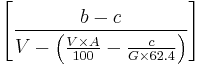$\left[ \frac{b - c} { V -\left(\frac{V \times A}{100} -\frac{c}{G \times 62.4}\right) }\right\rbrack\;$

Where:

M = Unit weight of air-free mortar, in pounds per cubic foot
b = Weight of concrete sample in unit weight container in pounds
c = Saturated surface-dry weight of aggregate retained on No. 4 sieve, in pounds
V = Volume of unit weight container, in cubic feet
A = Air content of concrete, in %, and
G = Specific gravity of coarse aggregate (Saturated surface-dry)

## 106.3.2.82.4 Test Differences

To determine compliance with the maximum permissible difference in results of those tests as shown above the following procedures are to be used:

Item 1, Weight per cubic foot calculated to an air-free basis

The procedure as shown above is to be followed on each of the two samples. The results are then to be compared and the difference between the two shown as the test result.

Example:
Weight per cubic foot, air-free
Sample A, 147.88 lb./ft.3
Sample B, 147.07 lb./ft.3
A (147.88) - B (147.07) = 0.8 lb./ft.3

Item 2. Air Content, percent by Volume of Concrete

The percent air content is to be determined on each of the two samples. The results are then to be compared and the difference between the two shown as the test result.

Example:
Air Content,
Sample A, 5.2 percent
Sample B, 5.0 percent
A (5.2) - B (5.0) = 0.2 percent

Item 3, Slump

The slump is to be determined on each of the two samples. The results are then to be compared and the difference between the two shown as the test result.

Example:
Slump
Sample A, 2 1/2 in.
Sample B, 2 1/4 in.
A (2 1/2) - B (2 1/4) = 1/4 in.

Item 4, Coarse Aggregate Content, portion by weight of each sample retained on No. 4 sieve

The procedure as shown above is to be followed on each of the two samples. The results are then to be compared and the difference between the two shown as the test result.

Example:
Coarse Aggregate Content
Sample A, 50.0 percent
Sample B, 47.1 percent
A (50.0) - (47.1) = 2.9 percent

Item 5, Unit Weight of Air-Free Mortar, based on average for all comparative samples tested

The procedure as shown above is to be followed on each of the two samples. The results of the two samples are then to be averaged. The percent difference between the two results is to be determined by dividing the difference between the two results by the average of the two results, multiplied by 100.

Example:
Unit Weight of Air-Free Mortar
Sample A, 137.58 lb./ft.3
Sample B, 136.06 lb./ft.3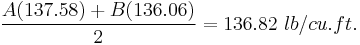$\frac{A (137.58) + B (136.06)}{2} = 136.82\ lb/cu.ft.$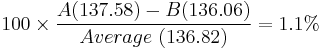$100 \times \frac{A (137.58) - B (136.06)}{Average\ (136.82)} = 1.1%$

Item 6, Average Compressive Strength at 7 days

The two compressive samples are to be prepared and tested as described above. The results of the three specimens comprising each sample are to be determined. The percent difference is then determined by dividing the difference between the average for each sample by the average for all specimens, multiplied by 100.

Example:
Sample A
Specimen 1, 3240 psi
Specimen 2, 3160 psi
Specimen 3, 3230 psi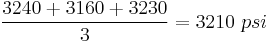$\frac{3240 + 3160 + 3230}{3} = 3210 \ psi$
Sample B
Specimen 4, 2900 psi
Specimen 5, 2980 psi
Specimen 6, 3100 psi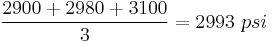$\frac{2900 + 2980 + 3100}{3} = 2993\ psi$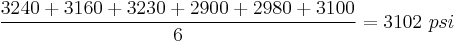$\frac{3240 + 3160 + 3230 + 2900 + 2980 + 3100}{6} = 3102\ psi$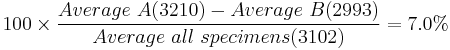$100\times \frac {Average\ A (3210) - Average\ B (2993)}{Average\ all\ specimens (3102)} = 7.0%$

For plants having more than one mixer drum, the prescribed tests may be performed on only one drum if the drums are made by the same manufacturer, are of the same size and are in comparable condition as to wear of blades, etc.